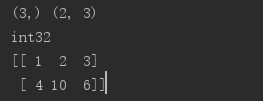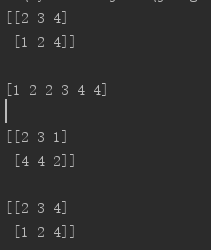• 利用python进行数据分析 Numpy Numpy简单使用（入门） Pandas入门 Pandas入门系列（一）-- Series Pandas入门系列（二）-- DataFrame Pandas入门系列（）-- 深入理解Series和DataFrame Pandas入门...


所有资料汇总学习:点这里
利用python进行数据分析
Numpy
Numpy简单使用（入门）
Pandas入门
Pandas入门系列（一）-- Series
Pandas入门系列（二）-- DataFrame
Pandas入门系列（三）-- 深入理解Series和DataFrame
Pandas入门系列（四） – Pandas io操作
Pandas入门系列（五） – Indexing和Selecting
Pandas入门系列（六) – reindex
Pandas入门系列（七) – NaN
Pandas入门系列（八) – 多级index
Pandas入门系列（九) – Map和replace
pandas进阶
Pandas玩转数据（一) – 简单计算
Pandas玩转数据（二) – Series和DataFrame排序
Pandas玩转数据（三) – DataFrame重命名
Pandas玩转数据（四) – DataFrame的merge
Pandas玩转数据（五) – Concatenate和Combine
Pandas玩转数据（六) – 通过apply对数据进行处理
Pandas玩转数据（七) – Series和DataFrame去重
Pandas玩转数据（八) – 时间序列简单操作
Pandas玩转数据（九) – 时间序列的采样和画图
Pandas玩转数据（十) – 数据分箱技术Binning
Pandas玩转数据（十一) – 数据分组技术Groupby
Pandas玩转数据（十二) – 数据聚合技术Aggregation
Pandas玩转数据（十三) – 透视表
Pandas玩转数据（十四) – 分组和透视功能实战
Matplotlib入门
Matplotlib简单画图(一) – plot
Matplotlib简单画图(二) – subplot
Matplotlib简单画图(三) – pandas绘图之Series
Matplotlib简单画图(四) – pandas绘图之DataFrame
Matplotlib简单画图(五) – 直方图和密度图
Matplotlib扩展之Seaborn
Seaborn简单画图(一) – 散点图
Seaborn简单画图(二) – 直方图和密度图
Seaborn简单画图(三) – 柱状图和热力图
Seaborn简单画图(四) – 设置图形显示效果
Seaborn简单画图(五) – Seaborn调色功能
获取数据的一个库Tushare
Tushare获取数据
简单实战
股票市场分析实战


展开全文• 1.数据收集 scrapy：网页采集、爬虫。 scrapy-redis：分布式爬虫。 selenium：web测试、仿真浏览器。 2.数据处理 beautifulsoup：网页解释，提供lxml的支持。...3.数据分析 numpy：基于矩阵的数学计
1.数据收集

scrapy：网页采集、爬虫。
scrapy-redis：分布式爬虫。
selenium：web测试、仿真浏览器。
2.数据处理

beautifulsoup：网页解释库，提供lxml的支持。
lxml：xml解释库。
xlrd：excel文件读取。
xlwt：excel文件写入。
slutls：excel文件简单格式修改。
pywin32：excel文件的读取写入及复杂格式定制。
Python-docx：Word文件的读取写入。
3.数据分析

numpy：基于矩阵的数学计算库。
pandas：基于表格的统计分析库。
scipy：科学计算库，支持高阶抽象和复杂模型。
statsmodels：统计建模和计量经济学工具包。
scikit-learn：机器学习工具库。
gensim：自然语言处理工具库。
jeba：中文分词工具库。
4.数据存储

MySQL-python：mysql的读写接口库。
mysqlclient：mysql的读写接口库。
SQLAlchemy：数据库的ORM封装。
pymysql：sqlserver读写接口库。
redis：redis的读写接口。
PyMongo：MongoDB的读写接口。
5.数据呈现

matplotlib：流行的数据可视化库。
seaborn：美观的数据可视化库，基于matplotlib。
6.工具辅助

jupyter：基于web的pythonIDE，常用于数据分析。
chardet：字符检查工具。
ConfigParser：配置文件读写支持。
requests：HTTP库，用于网络访问。

原创出处

展开全文• 这里快速学习一下Python 中一个非常重要的第三方库 NumPy。它不仅是 Python 中使用最多的第三方库，而且还是 SciPy、Pandas 等数据科学的基础库。它所提供的数据结构比 Python 自身的“更高级、更高效”，可以这么说...
这里快速学习一下Python 中一个非常重要的第三方库 NumPy。它不仅是 Python 中使用最多的第三方库，而且还是 SciPy、Pandas 等数据科学的基础库。它所提供的数据结构比 Python 自身的“更高级、更高效”，可以这么说，NumPy 所提供的数据结构是 Python 数据分析的基础。在Python 数组结构中的列表 list，它实际上相当于一个数组的结构。而 NumPy 中一个关键数据类型就是关于数组的，那为什么还存在这样一个第三方的数组结构呢？实际上，标准的 Python 中，用列表 list 保存数组的数值。由于列表中的元素可以是任意的对象，所以列表中 list 保存的是对象的指针。虽然在 Python 编程中隐去了指针的概念，但是数组有指针，Python 的列表 list 其实就是数组。这样如果我要保存一个简单的数组 [0,1,2]，就需要有 3 个指针和 3 个整数的对象，这样对于 Python 来说是非常不经济的，浪费了内存和计算时间。使用第三方库 NumPy让我们的的 Python 科学计算更高效！为什么要用 NumPy 数组结构而不是 Python 本身的列表 list？这是因为列表 list 的元素在系统内存中是分散存储的，而 NumPy 数组存储在一个均匀连续的内存块中。这样数组计算遍历所有的元素，不像列表 list 还需要对内存地址进行查找，从而节省了计算资源。另外在内存访问模式中，缓存会直接把字节块从 RAM 加载到 CPU 寄存器中。因为数据连续的存储在内存中，NumPy 直接利用现代 CPU 的矢量化指令计算，加载寄存器中的多个连续浮点数。另外 NumPy 中的矩阵计算可以采用多线程的方式，充分利用多核 CPU 计算资源，大大提升了计算效率。当然除了使用 NumPy 外，我们还需要一些技巧来提升内存和提高计算资源的利用率。一个重要的规则就是：避免采用隐式拷贝，而是采用就地操作的方式。举个例子，如果我想让一个数值 x 是原来的两倍，可以直接写成 x*=2，而不要写成 y=x*2。这样速度能快到 2 倍甚至更多。既然 NumPy 这么厉害，我们快速学习从哪儿入手学习呢？在 NumPy 里有两个重要的对象：ndarray（N-dimensional array object）解决了多维数组问题，而 ufunc（universal function object）则是解决对数组进行处理的函数。下面，我们来一一学习。ndarray 对象ndarray 实际上是多维数组的含义。在 NumPy 数组中，维数称为秩（rank），一维数组的秩为 1，二维数组的秩为 2，以此类推。在 NumPy 中，每一个线性的数组称为一个轴（axes），其实秩就是描述轴的数量。ndarray 对象是如何创建数组的，又是如何处理结构数组的呢？importnumpy as npa= np.array([1,2,3])b= np.array([[1,2,3],[4,5,6]])b[1,1] = 10print(a.shape,b.shape)print(a.dtype)print(b)结果如下：创建数组前，你需要引用 NumPy 库，可以直接通过 array 函数创建数组如果是多重数组，比如示例里的 b，那么该怎么做呢？你可以先把一个数组作为一个元素，然后嵌套起来。比如示例 b 中的 [1,2,3] 就是一个元素，然后 [4,5,6][7,8,9] 也是作为元素，然后把三个元素再放到 [] 数组里，赋值给变量 b。当然数组也是有属性的，比如你可以通过函数 shape 属性获得数组的大小，通过 dtype 获得元素的属性。如果你想对数组里的数值进行修改的话，直接赋值即可，注意下标是从 0 开始计的，所以如果你想对 b 数组，九宫格里的中间元素进行修改的话，下标应该是 [1,1]。结构数组如果你想统计一个班级里面学生的姓名、年龄，以及语文、英语、数学成绩该怎么办？当然你可以用数组的下标来代表不同的字段。比如下标为 0 的是姓名、小标为 1 的是年龄等，但是这样不显性。实际上在 C 语言里，可以定义结构数组，也就是通过 struct 定义结构类型，结构中的字段占据连续的内存空间，每个结构体占用的内存大小都相同，那在 NumPy 中是怎样操作的呢？importnumpy as nppersontype= np.dtype({'names':['name', 'age', 'chinese', 'math', 'english'],'formats':['S32','i', 'i', 'i', 'f']})peoples= np.array([("ZhangFei",32,75,100, 90),("GuanYu",24,85,96,88.5),("ZhaoYun",28,85,92,96.5),("HuangZhong",29,65,85,100)],dtype=persontype)ages= peoples[:]['age']chineses= peoples[:]['chinese']maths= peoples[:]['math']englishs= peoples[:]['english']print(np.mean(ages))print(np.mean(chineses))print(np.mean(maths))print (np.mean(englishs))这个例子，首先在 NumPy 中是用 dtype 定义的结构类型，然后在定义数组的时候，用 array 中指定了结构数组的类型 dtype=persontype，这样你就可以自由地使用自定义的 persontype 了。比如想知道每个人的语文成绩，就可以用 chineses = peoples[:]["chinese’]，当然 NumPy 中还有一些自带的数学运算，比如计算平均值使用 np.mean。ufunc 运算ufunc 是 universal function 的缩写，确如其名，它能对数组中每个元素进行函数操作。NumPy 中很多 ufunc 函数计算速度非常快，因为都是采用 C 语言实现的。连续数组的创建NumPy 可以很方便地创建连续数组，比如我使用 arange（注意这里最新版的numpy已经没有这个函数（被range取代了）） 或 linspace 函数进行创建importnumpy as npx1 = range(1,11,2)x2= np.linspace(1,9,5)print(x1,x2) #[1. 3. 5. 7. 9.]range 和 np.linspace 起到的作用是一样的，都是创建等差数组。这两个数组的结果 x1,x2 都是 [1 3 5 7 9]。结果相同，但是你能看出来创建的方式是不同的。内置函数 range()，通过指定初始值、终值、步长来创建等差数列的一维数组，默认是不包括终值的。linspace 是 linear space 的缩写，代表线性等分向量的含义。linspace() 通过指定初始值、终值、元素个数来创建等差数列的一维数组，默认是包括终值的。算数运算通过 NumPy 可以自由地创建等差数组，同时也可以进行加、减、乘、除、求 n 次方和取余数。importnumpy as npx1= range(1,11,2)x2= np.linspace(1,9,5)print(np.add(x1,x2))print(np.subtract(x1,x2))print(np.multiply(x1,x2))print(np.divide(x1,x2))print(np.power(x1,x2))print(np.remainder(x1,x2))以 x1, x2 数组为例，求这两个数组之间的加、减、乘、除、求 n 次方和取余数。在 n 次方中，x2 数组中的元素实际上是次方的次数，x1 数组的元素为基数在取余函数里，你既可以用 np.remainder(x1, x2)，也可以用 np.mod(x1, x2)，结果是一样的。统计函数当我们想要对一堆数据有更清晰的认识，就需要对这些数据进行描述性的统计分析，比如了解这些数据中的最大值、最小值、平均值，是否符合正态分布，方差、标准差多少等等。它们可以让你更清楚地对这组数据有认知。在 NumPy 中如何使用这些统计函数？计数组 / 矩阵中的最大值函数 amax()，最小值函数 amin()（注意是延着哪个轴！！！）importnumpy as npa= np.array([[1,2,3], [4,5,6], [7,8,9]])print(np.amin(a))      #1print (np.amin(a,0))   #[1 2 3]print (np.amin(a,1))   #[1 4 7]print (np.amax(a))     #9print (np.amax(a,0))   #[7 8 9]print (np.amax(a,1))   #[3 6 9]amin() 用于计算数组中的元素沿指定轴的最小值。对于一个二维数组 a，amin(a) 指的是数组中全部元素的最小值amin(a,0) 是延着 axis=0 轴的最小值（理解成横轴），axis=0 轴是把元素看成了 [1,4,7], [2,5,8], [3,6,9] 三个元素，所以最小值为 [1,2,3]amin(a,1) 是延着 axis=1 轴的最小值（理解成纵轴），axis=1 轴是把元素看成了 [1,2,3], [4,5,6], [7,8,9] 三个元素，所以最小值为 [1,4,7]同理amax() 是计算数组中元素沿指定轴的最大值。统计最大值与最小值之差 ptp()importnumpy as npa= np.array([[1,2,3], [4,5,6], [7,8,9]])print (np.ptp(a))      #8print (np.ptp(a,0))   #[6,6,6]print (np.ptp(a,1))   #[2,2,2]对于相同的数组 a，np.ptp(a) 可以统计数组中最大值与最小值的差，即 9-1=8。同样 ptp(a,0) 统计的是沿着 axis=0 轴的最大值与最小值之差，即 7-1=6（当然 8-2=6,9-3=6，第三行减去第一行的 ptp 差均为 6）ptp(a,1) 统计的是沿着 axis=1 轴的最大值与最小值之差，即 3-1=2（当然 6-4=2, 9-7=2，即第三列与第一列的 ptp 差均为 2）。统计数组的百分位数 percentile()importnumpy as npa= np.array([[1,2,3], [4,5,6], [7,8,9]])print (np.percentile(a, 50))          #5.0print (np.percentile(a, 50, axis=0))  #[4,5,6]print (np.percentile(a, 50, axis=1))  #[2,5,8]同样，percentile() 代表着第 p 个百分位数，这里 p 的取值范围是 0-100，如果 p=0，那么就是求最小值，如果 p=50 就是求平均值，如果 p=100 就是求最大值。同样你也可以求得在 axis=0 和 axis=1两个轴上的 p% 的百分位数。统计数组中的中位数 median()、平均数 mean()importnumpy as npa= np.array([[1,2,3], [4,5,6], [7,8,9]])#求中位数print (np.median(a))         #5.0print (np.median(a, axis=0)) #[4. 5. 6.]print (np.median(a, axis=1)) #[2. 5. 8.]#求平均数print (np.mean(a))          #5.0print (np.mean(a, axis=0))  #[4. 5. 6.]print (np.mean(a, axis=1))  #[2. 5. 8.]用 median() 和 mean() 求数组的中位数、平均值，同样也可以求得在 axis=0 和 1 两个轴上的中位数、平均值。统计数组中的加权平均值 average()importnumpy as npa= np.array([1,2,3,4])wts= np.array([1,2,3,4])print (np.average(a))               #2.5print (np.average(a,weights=wts))   #3.0average() 函数可以求加权平均，加权平均的意思就是每个元素可以设置个权重，默认情况下每个元素的权重是相同的所以 np.average(a)=(1+2+3+4)/4=2.5，你也可以指定权重数组 wts=[1,2,3,4]，这样加权平均 np.average(a,weights=wts)=(1*1+2*2+3*3+4*4)/(1+2+3+4)=3.0。统计数组中的标准差 std()、方差 var()importnumpy as npa= np.array([1,2,3,4])print(np.std(a),np.var(a)) #1.118033988749895 1.25方差的计算是指每个数值与平均值之差的平方求和的平均值，即 mean((x - x.mean())** 2)。标准差是方差的算术平方根。在数学意义上，代表的是一组数据离平均值的分散程度。所以 np.var(a)=1.25, np.std(a)=1.118033988749895。NumPy 排序排序是算法中使用频率最高的一种，也是在数据分析工作中常用的方法，在大学期间的算法是（数据结构）课中学习（排序是必修课）。那么这些排序算法在 NumPy 中实现起来其实非常简单，一条语句就可以搞定。这里你可以使用 sort 函数，sort(a, axis=-1, kind="quicksort’, order=None)默认情况下使用的是快速排序；在 kind 里，可以指定 quicksort、mergesort、heapsort 分别表示快速排序、合并排序、堆排序。同样 axis 默认是 -1，即沿着数组的最后一个轴进行排序，也可以取不同的 axis 轴，或者 axis=None代表采用扁平化的方式作为一个向量进行排序。另外 order 字段，对于结构化的数组可以指定按照某个字段进行排序。importnumpy as npa= np.array([[4,3,2],[2,4,1]])print(np.sort(a))print()print (np.sort(a, axis=None))print()print (np.sort(a, axis=0))print()print (np.sort(a, axis=1))总结在 NumPy 学习中，重点要掌握的就是对数组的使用，因为这是 NumPy 和标准 Python 最大的区别。在 NumPy 中重新对数组进行了定义，同时提供了算术和统计运算，你也可以使用 NumPy 自带的排序功能，一句话就搞定各种排序算法。当然要理解 NumPy 提供的数据结构为什么比 Python 自身的“更高级、更高效”，要从对数据指针的引用角度进行理解。
展开全文• Python数据分析常用 Numpy, Scipy, Matplotlib, Pandas, Scikit-Learn, Keras, Gensim. 通过pip安装 # 以numpy为例 pip install numpy Numpy 提供多维数组功能，以及对数据进行快速处理的函数。 Scipy 提供矩阵...
Python数据分析常用库
Numpy, Scipy, Matplotlib, Pandas, Scikit-Learn, Keras, Gensim.

通过pip安装
# 以numpy为例
pip install numpy

Numpy
提供多维数组功能，以及对数据进行快速处理的函数。

Scipy
提供矩阵，以及大量基于矩阵运算的对象和函数。
功能：最优化、线性代数、积分、插值、拟合、信号处理和图像处理、常微分方程求解等。

参考链接：
http//www.scipy.org/
Matplotlib
数据可视化，主要用于二维绘图。

作图基本代码：
import matplotlib.pyplot as plt

# 设置图像大小
plt.figure(figsize=(10, 15))
# 作图，设置标签、线条颜色、线条大小，线条类型
plt.plot(x, y, 'b--', label = 'example', linewidth = 2)
# color = 'red'
# x轴和y轴名称
plt.xlabel('Time(s)')
plt.ylabel('Volumn')
# 标题
plt.title('A Simple Example')
# y轴的显示范围
plt.ylim(0, 2.2)
# 显示图例
plt.legend()
#显示作图结果
plt.show() # 只需要打一次

参考链接：
http://matplotlib.org/
Seaborn
	基于matplotlib的一种视觉可视化工具。
里面有countplot，barplot，heatmap，distplot，pairplot

参考链接：https://seaborn.pydata.org/
Pandas
是python中最强大的数据分析和探索工具。支持类似于SQL的数据增删改查；支持时间序列分析；支持灵活处理缺失值等。
参考《利用python进行数据分析》
基本的数据结构是：Series，DataFrame

import pandas as pd

# 读取文件（存储路径不能带中文）
pd.read_excel('data.xls')
pd.read_csv('data.csv', encoding = 'utf-8')
# 读取文本格式的数据一般用encoding指定编码

参考链接：
http://pandas.pydata.org/pandas-docs/stable/
StatsModels
注重数据的统计建模分析，更有R语言的味道。

参考链接：
http://statsmodels.sourceforge.net/stable/index.html
Scikit-Learn
与机器学习相关的库。它提供完善的机器学习工具箱，包括数据预处理、分类、回归、聚类、预测和模型分析等。
提前先安装好相关库：Numpy，Scipy，Matplotlib。

参考链接：
http://scikit-learn.org/
Keras
包含人工神经网络模型。用Keras搭建神经网络。

Gensim
用来处理语言方面的任务：文本相似度、LDA、Word2Vec等。



展开全文• python适合数据分析类的工作是众所周知的，那么金融数据是其中重要的一个部分，国内这样提供免费的金融数据的API并不是很多，可能是我孤陋寡闻，有更好的选择还请各位大神多多指教~~ Tushare简单的介绍：Tushare是...
• 第三方库 Numpy——提供数组支持，以及相应的高效的处理函数 Scipy——提供矩阵支持，以及矩阵相关的数值计算模块 Matplotlib——数据可视化工具 Pandas——数据分析和探索工具 StasModels——统计建模 Scikit...
• install python ...对于想要用Python进行数据分析，就需要强大的Python第三库如numpy、pandas、matplotlib、scipy必不可少，而Python本身除标准库外都需要另行安装，安装这些第三方库（包）成为许多人的问题，...
• 易知大学任务（2）成绩表雷达分析图概述Numpy 最基本的库，是用于处理含有同种元素的多维数组运算的第三方库—科学计算包，python数据分析及科学计算的基础库，几乎支撑所有其他库—支持N维数组运算、处理大型矩阵、...
• python的使用中，经常会遇到各种环境的安装包问题，大部分安装包都可以直接用pip的方式安装，但是有时候pip安装会很慢，有时候会导致安装失败，这个链接里面对应的python第三方库比较全：https://www.lfd.uci.edu/...pip
• 下面简单介绍下网络爬虫、自动化、数据分析与可视化、WEB开发、机器学习和其他常用的一些第三方库，如果有你感兴趣的库，不妨去试试它的功能吧。1、网络爬虫•requests-对HTTP协议进行高度封装，支持非常丰富的链接...
• ## python的重要第三方库你用过多少？

千次阅读 多人点赞 2021-04-23 20:39:57
python 能被称作最方便的语言不是没有道理的它的第三方库功能强大到毁天灭地（开个玩笑） 下面就介绍几个特别好用的第三方库给大家提个方向，想详细研究的小伙伴可以到官网查查那么开始。 Python库大全网络爬虫...
• 1.数据收集 scrapy：网页采集、爬虫。 scrapy-redis：分布式爬虫。 selenium：web测试、仿真浏览器。 2.数据处理 ...beautifulsoup：网页解释，提供lxml的支持。...3.数据分析 numpy：基于矩阵的数学计算。 pa
• 其中既有Python内置函数和标准库，又有第三方库和工具。这些库可用于文件读写、网络抓取和解析、数据连接、数清洗转换、数据计算和统计分析、图像和视频处理、音频处理、数据挖掘/机器学习/深度学习、数据可视化、...
• 其中既有Python内置函数和标准库，又有第三方库和工具。这些库可用于文件读写、网络抓取和解析、数据连接、数清洗转换、数据计算和统计分析、图像和视频处理、音频处理、数据挖掘/机器学习/深度学习、数据可视化、...
• Python常用第三方库一、 文件读写二、网络抓取和解析三、数据库连接四、数据清洗转换五、数据计算和统计分析六、自然语言处理和文本挖掘七、图像和视频处理八、音频处理九、数据挖掘/机器学习/深度学习十、数据可视...
• 对于想要用Python进行数据分析，就需要强大的Python第三库如numpy、pandas、matplotlib、scipy必不可少，而Python本身除标准库外都需要另行安装，安装这些第三方库（包）成为许多人的问题，下面我们以安装requests包...
• Python数据分析基础入门（二）python数据分析第三方库介绍NumpyScipyPandasScikit-Learn python数据分析第三方库介绍 Python本身数据分析功能不强，需要安装一些第三方库来增强它的能力，一般用的的库有Numpy、Scipy...
• pandas是python优秀的第三方库，提供高性能的数据类型和分析工具。pandas基于numpy实现，常与numpy与matplotlib库一起使用。 import pandas as pd 总的来说pandas有两个基本的数据类型：Series和DataFrame 争...
• 在做数据处理和数据分析的时候，我们总是少不了测试数据，比如你想对设一个合理的数据清洗方案，但有的时候事先能够找一些样例做测试，但大多数的情况是我们自己构建一个测试数据。但对于我这个选择困难症的人，每次...
• Python第三方库outline 强大广泛丰富的第三方库Python获得如此的成功，相比其他语言，不重复造轮子。 在数据科学领域，Python，R语言是最主流的语言，金融领域MATLAB也已经逐渐退出，更多的拥抱Python。 outline：...
• 其中既有Python内置函数和标准库，又有第三方库和工具。这些库可用于文件读写、网络抓取和解析、数据连接、数清洗转换、数据计算和统计分析、图像和视频处理、音频处理、数据挖掘/机器学习/深度学习、数据可视化、...
• 目录前言Python保留字基本数据类型整数类型浮点数类型复数类型字符串类型字符串的格式化组合数据类型集合类型序列类型元组类型列表...第三方库从数据处理到人工智能Python数据分析Python数据可视化Python文本处理Pyt
• 目录1 Pandas介绍2 Pandas库的Series类型3 Pandas库的...Pandas是Python第三方库，提供高性能易用数据类型和分析工具。 Pandas基于Numpy实现，常与Numpy和Matplotlib一同使用。 Pandas的引用： import pandas as ...
• 第三方库扩展（以vs2017下安装pandas为例） Reference：https://blog.csdn.net/weixin_40184249/article/details/80720015 工具—PythonPython 环境—在PowerShell 中打开 pip install --index ...vs2017 数组 矩阵
• 数据分析方向 numpy pandas scipy 文本处理方向 pdfminer python-docx beautifuisoup4 数据可视化方向 matplotlib seaborn mayavi 用户图形界面方向 PyQt5 wxPython PyGTK 机器学习方向 scikit -learn TebsorFlow mx...
• 本文章主要介绍了一些优秀的Python第三方库，其中包括：从数据处理到人工智能；从web解析到网络空间等等 Python库之数据分析 ​​​​​​​ Python库之数据可视化 ​​​​​​​ Python库之文本处理 ​...人工智能 网站开发
• 对于想要用Python进行数据分析，就需要强大的Python第三库如numpy、pandas、matplotlib、scipy必不可少，而Python本身除标准库外都需要另行安装，安装这些第三方库（包）成为许多人的问题，下面我们以安装requests.....linux...

# python数据分析第三方库python 订阅